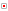home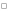member logincontact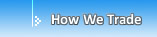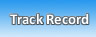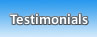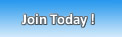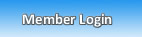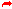Recent Trading Results

Conservative Alpha Portfolio

Mar 2011 = 4.8% gain
Feb 2011 = 2.9% gainJan 2011 = 4.1% gain
Dec 2010 = 4.9% gain
Nov 2010 = 6.2% gain
Oct 2010 = 7.1% gain
Sept 2010 = 11.6% gain
Aug 2010 = 13.2% gain
July 2010 = 6.2% gain
June 2010 = 11.8% gain
May 2010 = 15.2% gain

Moderate Beta Portfolio

Mar 2011 = 4.6% gain
Feb 2011 = 3.9% gain
Jan 2011 = 6.1% gain
Dec 2010 = 5.9% gain
Nov 2010 = 7.1% gain
Oct 2010 = 6.2% gain
Sept 2010 = 23.4% gain
Aug 2010 = 22.3% gain
July 2010 = 17.7% gain
June 2010 = 29.2% gain
May 2010 = 3.2% loss

Aggressive Trades

AAPL +314%
RIMM +97%
VMW +451%
BAC +77%

 Winning With Options - Track Record

Please fill out the form below and you will receive our impressive - long term - track record in PDF format in an email sent directly to you!

 First name*: Last name: E-mail*:

 Copyright © 2006-2010 Winning With Options. All Rights Reserved.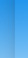Terms & Conditions | Privacy Policy